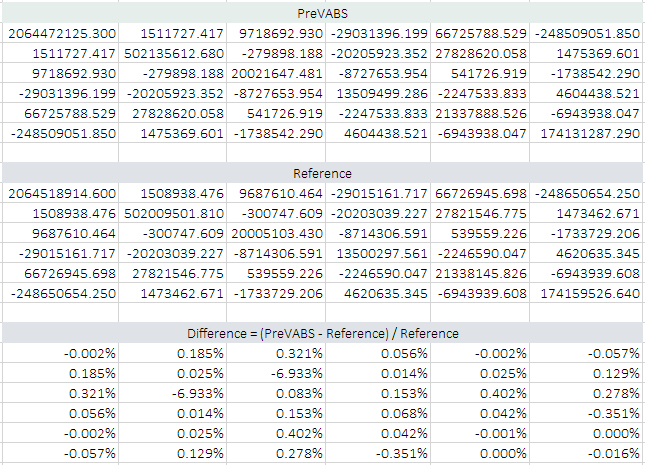# Airfoil (MH-104)#

## Problem description#

This example demonstrates the capability of building a cross section having an airfoil shape, which is commonly seen on wind turbine blades or helicopter rotor blades. This example is also studied in [CHEN2010]. A sketch of a cross section for a typical wind turbine blade is shown in Fig. 59. The airfoil is MH 104 (http://m-selig.ae.illinois.edu/ads/coord_database.html#M). In this example, the chord length $$CL=1.9$$ m. The origin O is set to the point at 1/4 of the chord. Twist angle $$\theta$$ is $$0^\circ$$. There are two webs, whose right boundaries are at the 20% and 50% location of the chord, respectively. Both low pressure and high pressure surfaces have four segments. The dividing points between segments are listed in Table 54. Materials are given in Table 55 and layups are given in Table 56. A complete $$6\times 6$$ stiffness matrix is given in Table 57. Complete input files can be found in examples\ex_airfoil\, including mh104.xml, basepoints.dat, baselines.xml, materials.xml, and layups.xml.

Table 54 Dividing points#

Between segments

Low pressure surface

High pressure surface

$$(x, y)$$

$$(x, y)$$

1 and 2

(0.004053940, 0.011734800)

(0.006824530, -0.009881650)

2 and 3

(0.114739930, 0.074571970)

(0.126956710, -0.047620490)

3 and 4

(0.536615950, 0.070226120)

(0.542952100, -0.044437080)

Table 55 Material properties#

Name

Type

Density

$$E_{1}$$

$$E_{2}$$

$$E_{3}$$

$$G_{12}$$

$$G_{13}$$

$$G_{23}$$

$$\nu_{12}$$

$$\nu_{13}$$

$$\nu_{23}$$

$$10^3\ \mathrm{kg/m^3}$$

$$\mathrm{GPa}$$

$$\mathrm{GPa}$$

$$\mathrm{GPa}$$

$$\mathrm{GPa}$$

$$\mathrm{GPa}$$

$$\mathrm{GPa}$$

Uni-directional FRP

orthotropic

1.86

37.00

9.00

9.00

4.00

4.00

4.00

0.28

0.28

0.28

Double-bias FRP

orthotropic

1.83

10.30

10.30

10.30

8.00

8.00

8.00

0.30

0.30

0.30

Gelcoat

orthotropic

1.83

1e-8

1e-8

1e-8

1e-9

1e-9

1e-9

0.30

0.30

0.30

Nexus

orthotropic

1.664

10.30

10.30

10.30

8.00

8.00

8.00

0.30

0.30

0.30

Balsa

orthotropic

0.128

0.01

0.01

0.01

2e-4

2e-4

2e-4

0.30

0.30

0.30

Table 56 Layups#

Name

Layer

Material

Ply thickness

Orientation

Number of plies

$$\mathrm{m}$$

$$\circ$$

layup_1

1

Gelcoat

0.000381

0

1

2

Nexus

0.00051

0

1

3

Double-bias FRP

0.00053

20

18

layup_2

1

Gelcoat

0.000381

0

1

2

Nexus

0.00051

0

1

3

Double-bias FRP

0.00053

20

33

layup_3

1

Gelcoat

0.000381

0

1

2

Nexus

0.00051

0

1

3

Double-bias FRP

0.00053

20

17

4

Uni-directional FRP

0.00053

30

38

5

Balsa

0.003125

0

1

6

Uni-directional FRP

0.00053

30

37

7

Double-bias FRP

0.00053

20

16

layup_4

1

Gelcoat

0.000381

0

1

2

Nexus

0.00051

0

1

3

Double-bias FRP

0.00053

20

17

4

Balsa

0.003125

0

1

5

Double-bias FRP

0.00053

0

16

layup_web

1

Uni-directional FRP

0.00053

0

38

2

Balsa

0.003125

0

1

3

Uni-directional FRP

0.00053

0

38

## Result#

 $$\phantom{-}2.395\times 10^9$$ $$\phantom{-}1.588\times 10^6$$ $$\phantom{-}7.215\times 10^6$$ $$-3.358\times 10^7$$ $$\phantom{-}6.993\times 10^7$$ $$-5.556\times 10^8$$ $$\phantom{-}1.588\times 10^6$$ $$\phantom{-}4.307\times 10^8$$ $$-3.609\times 10^6$$ $$-1.777\times 10^7$$ $$\phantom{-}1.507\times 10^7$$ $$\phantom{-}2.652\times 10^5$$ $$\phantom{-}7.215\times 10^6$$ $$-3.609\times 10^6$$ $$\phantom{-}2.828\times 10^7$$ $$\phantom{-}8.440\times 10^5$$ $$\phantom{-}2.983\times 10^5$$ $$-5.260\times 10^6$$ $$-3.358\times 10^7$$ $$-1.777\times 10^7$$ $$\phantom{-}8.440\times 10^5$$ $$\phantom{-}2.236\times 10^7$$ $$-2.024\times 10^6$$ $$\phantom{-}2.202\times 10^6$$ $$\phantom{-}6.993\times 10^7$$ $$\phantom{-}1.507\times 10^7$$ $$\phantom{-}2.983\times 10^5$$ $$-2.024\times 10^6$$ $$\phantom{-}2.144\times 10^7$$ $$-9.137\times 10^6$$ $$-5.556\times 10^8$$ $$\phantom{-}2.652\times 10^5$$ $$-5.260\times 10^6$$ $$\phantom{-}2.202\times 10^6$$ $$-9.137\times 10^6$$ $$\phantom{-}4.823\times 10^8$$
 $$\phantom{-}2.389\times 10^9$$ $$\phantom{-}1.524\times 10^6$$ $$\phantom{-}6.734\times 10^6$$ $$-3.382\times 10^7$$ $$-2.627\times 10^7$$ $$-4.736\times 10^8$$ $$\phantom{-}1.524\times 10^6$$ $$\phantom{-}4.334\times 10^8$$ $$-3.741\times 10^6$$ $$-2.935\times 10^5$$ $$\phantom{-}1.527\times 10^7$$ $$\phantom{-}3.835\times 10^5$$ $$\phantom{-}6.734\times 10^6$$ $$-3.741\times 10^6$$ $$\phantom{-}2.743\times 10^7$$ $$-4.592\times 10^4$$ $$-6.869\times 10^2$$ $$-4.742\times 10^6$$ $$-3.382\times 10^7$$ $$-2.935\times 10^5$$ $$-4.592\times 10^4$$ $$\phantom{-}2.167\times 10^7$$ $$-6.279\times 10^4$$ $$\phantom{-}1.430\times 10^6$$ $$-2.627\times 10^7$$ $$\phantom{-}1.527\times 10^7$$ $$-6.869\times 10^2$$ $$-6.279\times 10^4$$ $$\phantom{-}1.970\times 10^7$$ $$\phantom{-}1.209\times 10^7$$ $$-4.736\times 10^8$$ $$\phantom{-}3.835\times 10^5$$ $$-4.742\times 10^6$$ $$\phantom{-}1.430\times 10^6$$ $$\phantom{-}1.209\times 10^7$$ $$\phantom{-}4.406\times 10^8$$

Note

The errors between the result and the reference are caused by the difference of modeling of the trailing edge. If reduce the trailing edge skin to a single thin layer, then the difference between the trailing edge shapes is minimized, and the two resulting stiffness matrices are basically the same, as shown in Fig. 64.Figure 64 Comparison of stiffness matrices after modifying the trailing edge.#